### IMO Shortlist 2010 problem G1

Kvaliteta:
Avg: 3.5
Težina:
Avg: 5.7
Let$ABC$ be an acute triangle with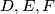$D, E, F$ the feet of the altitudes lying on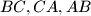$BC, CA, AB$ respectively. One of the intersection points of the line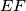$EF$ and the circumcircle is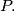$P.$ The lines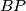$BP$ and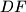$DF$ meet at point$Q.$ Prove that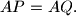$AP = AQ.$

Proposed by Christopher Bradley, United Kingdom
Source: Međunarodna matematička olimpijada, shortlist 2010